## Clark's TriangleA Number Triangle created by setting the Vertex equal to 0, filling one diagonal with 1s, the other diagonal with multiples of an Integer, and filling in the remaining entries by summing the elements on either side from one row above. Call the first columnand the last columnso that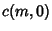(1)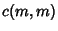(2)

then use the Recurrence Relation(3)

to compute the rest of the entries. For, we have(4)(5)

For arbitrary, the value can be computed by Summing this Recurrence,(6)

Now, forwe have(7)(8)

so Summing the Recurrence gives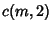(9)

Similarly, forwe have(10)

Taking the Sum,(11)

Evaluating the Sum gives(12)

So far, this has just been relatively boring Algebra. But the amazing part is that ifis chosen as the Integer, thenandsimplify to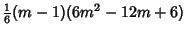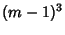(13)(14)

which are consecutive Cubesand nonconsecutive Squares.

See also Bell Triangle, Catalan's Triangle, Euler's Triangle, Leibniz Harmonic Triangle, Number Triangle, Pascal's Triangle, Seidel-Entringer-Arnold Triangle, Sum

References

Clark, J. E. Clark's Triangle.'' Math. Student 26, No. 2, p. 4, Nov. 1978.